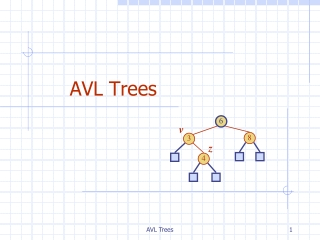DownloadDownload PresentationAVL Trees

# AVL Trees

Télécharger la présentation## AVL Trees

- - - - - - - - - - - - - - - - - - - - - - - - - - - E N D - - - - - - - - - - - - - - - - - - - - - - - - - - -
##### Presentation Transcript

1. 6 v 8 3 z 4 AVL Trees AVL Trees

2. Balanced Binary Tree • There are many kinds of balanced tree • The definition of balanced binary tree • A binary tree with height O(log n) or exactly log n AVL Trees

3. AVL Tree Definition • AVL trees are balanced. • An AVL Tree is a binary search tree such that for every internal node v of T, the heights of the children o f v can differ by at most 1. An example of an AVL tree where the heights are shown next to the nodes: AVL Trees

4. n(2) 3 n(1) 4 Height of an AVL Tree • Fact: The height of an AVL tree storing n keys is O(log n). • Proof: Let us bound n(h): the minimum number of internal nodes of an AVL tree of height h. • We easily see that n(1) = 1 and n(2) = 2 • For n > 2, an AVL tree of height h contains the root node, one AVL subtree of height h-1 and another of height h-2. • That is, n(h) = 1 + n(h-1) + n(h-2) • Knowing n(h-1) > n(h-2), we get n(h) > 2n(h-2). So n(h) > 2n(h-2), n(h) > 4n(h-4), n(h) > 8n(n-6), … (by induction), n(h) > 2in(h-2i) • Solving the base case we get: n(h) > 2 h/2-1 • Taking logarithms: h < 2log n(h) +2 • Thus the height of an AVL tree is O(log n) AVL Trees

5. 44 17 78 32 50 88 48 62 Insertion in an AVL Tree • Insertion is as in a binary search tree • Always done by expanding an external node. • Example: 44 17 78 32 50 88 48 62 54 Inserted node before insertion after insertion AVL Trees

6. 44 17 78 32 50 88 48 62 54 Insertion in an AVL Tree • Let • z be the first unbalanced node encountered while traveling up the tree from w. • y be the child of z with the larger height, and • xbe the child of y with the larger height. c=z a=y b=x w AVL Trees

7. a=z a=z T0 c=y T0 b=y b=x T3 c=x T1 b=y b=x T1 T2 T2 T3 a=z c=x a=z c=y T2 T3 T1 T3 T0 T0 T2 T1 Trinode Restructuring • let (a,b,c) be an inorder listing of x, y, z • perform the rotations needed to make b the topmost node of the three Case 2: double rotation (a right rotation about c, then a left rotation about a) (other two cases are symmetrical) Case 1: single rotation (a left rotation about a) AVL Trees

8. c = z b = y single rotation b = y a = x c = z a = x T T 3 0 T T T T T 0 2 1 2 3 Restructuring (as Single Rotations) • Single Rotations: AVL Trees T 1

9. a = z b = x a = z b = x c = y c = y c = y a = z b = x T T T 0 2 2 T T T T T T T T 3 0 1 3 0 1 3 2 T 1 Restructuring (as Double Rotations) • double rotations: double rotation AVL Trees

10. Restructuring (as Double Rotations) • double rotations: AVL Trees

11. T T 1 1 Insertion Example, continued unbalanced... 4 44 x 3 2 17 62 z y 2 1 2 78 32 50 1 1 1 ...balanced 54 88 48 T 2 T T AVL Trees 0 3

12. 44 17 62 32 50 78 88 48 54 Removal in an AVL Tree • Removal begins as in a binary search tree, which means the node removed will become an empty external node. Its parent, w, may cause an imbalance. • Example: 44 17 62 50 78 88 48 54 before deletion of 32 after deletion AVL Trees

13. Rebalancing after a Removal • Let z be the first unbalanced node encountered while travelling up the tree from w. Also, let y be the child of zwith the larger height, and let x be the child of y with the larger height. • We perform restructure(x) to restore balance at z. • As this restructuring may upset the balance of another node higher in the tree, we must continue checking for balance until the root of T is reached 62 44 a=z 44 78 17 62 w b=y 17 50 88 50 78 c=x 48 54 88 48 54 AVL Trees

14. Running Times for AVL Trees • a single restructure is O(1) • using a linked-structure binary tree • find is O(log n) • height of tree is O(log n), no restructures needed • insert is O(log n) • initial find is O(log n) • Restructuring up the tree, maintaining heights is O(log n) • remove is O(log n) • initial find is O(log n) • Restructuring up the tree, maintaining heights is O(log n) AVL Trees